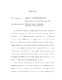## MONGE–AMPERE ITERATION2017
##### Authors
Hunter, Ryan
In this thesis we introduce a Monge–Ampere iteration to be a sequence of convex functions {ϕi}i∈N which solve the sequence of Monge–Ampere equations det(∇2ϕi+1) = h ◦ ϕi with second boundary values ∇ϕi+1(Rn) = A where h is a function of one variable and A is a bounded, convex set. Our main analytic theorem gives suﬃcient conditions on the function h and the set A so that a Monge– Ampere iteration {ϕi}, once correctly normalized, converges smoothly on compact sets to a convex solution of the Monge–Ampere equation det(∇2ϕ) = h ◦ ϕ with second boundary value ∇ϕ(Rn) = A. Monge–Ampere iterations {ϕi}i∈N arise as a sequence of solutions to optimal transport problems, so our convergence result can be interpreted as breaking apart the Monge–Ampere equation det(∇2ϕ) = h◦ϕ, ∇ϕ(Rn) = A into a sequence of optimal transport problems. We then turn to two geometric applications of our main theorem. The ﬁrst application, when h(t) = e−t, is to Ricci iteration, which was introduced by Rubinstein. We prove a sequence {ωi}i∈N of toric Ka¨hler metrics with ﬁxed edge singularities solving the K¨ahler–Ricci iteration on a toric Fano manifold converges, after being twisted by automorphisms, to a Ka¨hler–Einstein metric with the same singularities. This extends the smooth K¨ahler–Ricci iteration convergence theorem of Darvas–Rubinstein  to edge metrics on toric Fano manifolds. The second geometric application, when h(t) = t−(n+2), is to aﬃne diﬀerential geometry. We introduce the aﬃne iteration to be a sequence of graph immersions fi : Rn ,→ Rn+1 such that the aﬃne normal at fi+1(x) is a constant multiple of the position vector fi(x). Thus, the aﬃne iteration is a sequence of prescribed aﬃne normal problems. We prove for any aﬃne iteration {fi}i∈N there exists a sequence of matrices Mi ∈ Sln+1R such that {Mi ·fi(Rn)} converges smoothly to an aﬃne sphere.